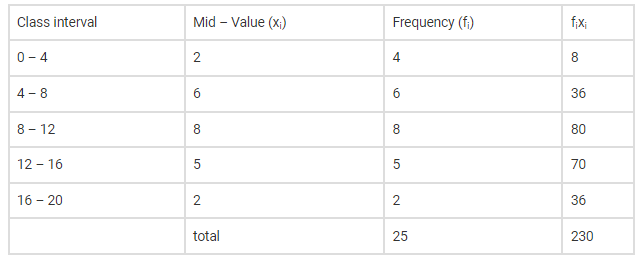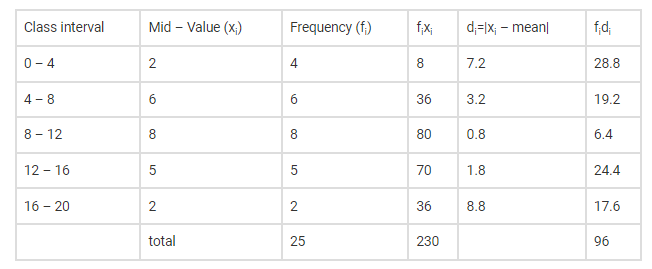# Calculate the mean deviation about the mean

Question:

Calculate the mean deviation about the mean for the following frequency distribution:Solution:

Given the frequency distribution

Now we have to find the mean deviation about the mean

Let us make a table of the given data and append other columns after calculationsHere mean,

$\overline{\mathrm{X}}=\frac{\sum \mathrm{f}_{\mathrm{i}} \mathrm{x}_{\mathrm{i}}}{\sum \mathrm{f}_{\mathrm{i}}}=\frac{230}{25}=9.2$

Now we have to find mean deviationHence Mean Deviation becomes,

$M . D=\frac{\sum f_{i} d_{i}}{\sum f_{i}}=\frac{96}{25}=3.84$

Therefore, the mean deviation about the mean of the distribution is 3.84